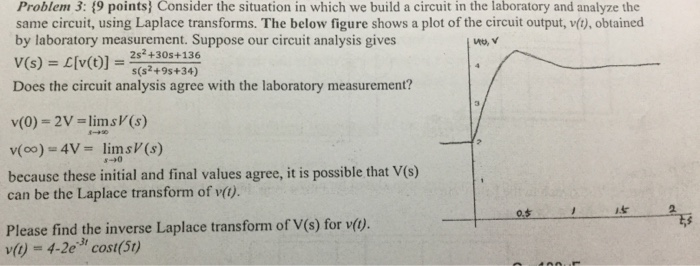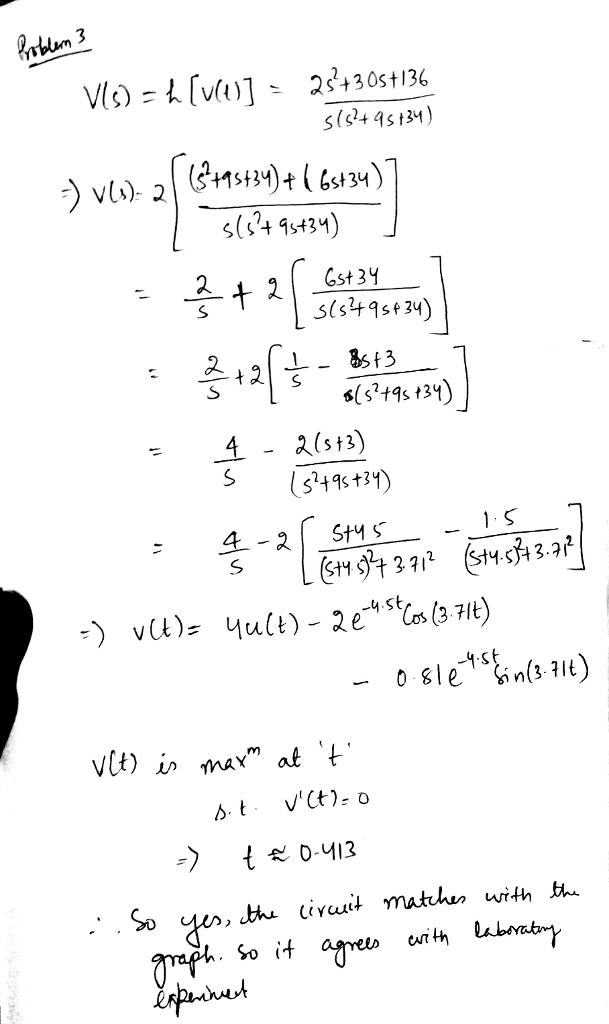# Question & Answer: Consider the situation in which we build a circuit in the laboratory and analyze the same circuit, using Laplace…..

Consider the situation in which we build a circuit in the laboratory and analyze the same circuit, using Laplace transforms. The below figure shows a plot of the circuit output, v(t), obtained by laboratory measurement. Suppose our circuit analysis gives V(s) = L[v(t)] = 2s^2 + 30s + 136/s (s^2 + 9s + 34) Does the circuit analysis agree with the laboratory measurement? v(0) = 2V = lim_x rightarrow s V (s) v(infinity) = 4V = lim_s rightarrow 0 s V(s) because these initial and final values agree, it is possible that V(s) can be the Laplace transform of v(t). Please find the inverse Laplace transform of V(s) for v(t). v(t) = 4 – 2e^-3t cos (5t)